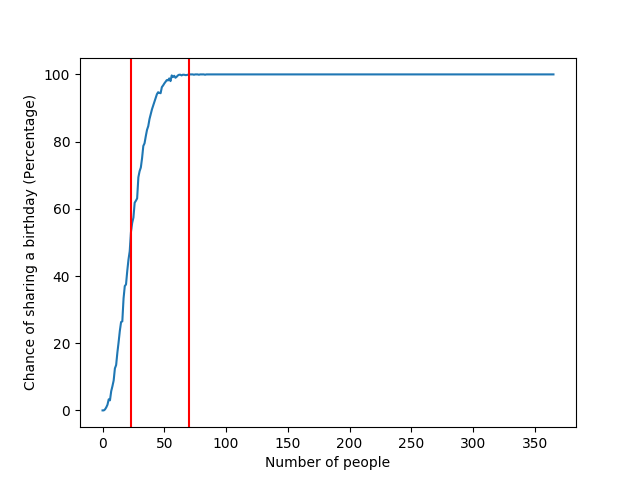# birthday

## The Birthday Paradox – The Proof is in the Python

This month is my wife’s, mother’s and my own birthday, all within a span of nine days. What are the odds of that? No idea, I’m no statistician. However, as a developer, I thought it would be fun to prove (or disprove?) the Birthday Paradox with some Python coding.If you haven’t heard of the Birthday Paradox, it states that as soon as you have 23 random people in a room, there is a 50 percent chance two of them have the same birthday. Once the number of people in the room is at least 70, there is a 99.9 percent chance. It sound counter intuitive as it takes a full 366 (a full year + 1) people to have a 100% chance.

So instead of doing this from the statistical side, let’s do it from the experimentation angle. We are going to generate 23 random days in a year and see if there is a duplicate day.

First, we create a function that will return a list of N number of `datetime` objects within a given year. We will then use that list to see if there are duplicates within it. In the first case, with 23 people, exactly half the time we run this function there should be at least one duplicate date.

```from random import randint
from datetime import datetime, timedelta

def random_birthdays(number_of_people):
first_day_of_year = datetime(2017, 1, 1)
return [first_day_of_year + timedelta(days=randint(0, 365))
for _ in range(number_of_people)]
```

We need to run this function a lot of times to get an average of how often there is a duplicate.  We’ll create a function that runs it a thousand times and returns percentage average of duplicate found. (As a percentage is between 0 and 1, such as .50, we are multiplying it by 100 so that .5 looks like “50% chance” later.)

```def determine_probability(number_of_people, run_amount=1_000):
dups_found = 0
for _ in range(run_amount):
birthdays = random_birthdays(number_of_people)
duplicates = set(x for x in birthdays if birthdays.count(x) > 1)
if len(duplicates) >= 1:
dups_found += 1

return dups_found/run_amount * 100```

Feel free to run it even more times than a 1000 for higher accuracy, max I tried was 100,000 which did have the most consistent results.

Finally, we add that last bit of code that actually calls the function and prints our findings.

```if __name__ == '__main__':
msg = ("{people} Random people have a {chance:.1f}%"
" chance of having a birthday on the same day")
for people in (23, 70):
print(msg.format(people=people, chance=determine_probability(people)))```

And wouldn’t ya know it, those fancy statisticians seem to be right.

23 Random people have a 50.4% chance of having a birthday on the same day
70 Random people have a 99.9% chance of having a birthday on the same day

At the top of the post, you saw a plot generated by calculating the first 100 people’s worth of probabilities, with red vertical markers at 23 and 70.  It is rather smooth due to increasing the `run_amount` to 10,000. Below is a plot of an entire 366 people, but only a 1000 runs per number of people.To accomplish this, I used `matplotlib` and mixed in some multiprocessing to speed up the probability generation. Here is the final entire file.

```from random import randint
from datetime import datetime, timedelta
from multiprocessing import Pool, cpu_count

import matplotlib.pyplot as plt

def random_birthdays(number_of_people):
first_day_of_year = datetime(2017, 1, 1)
return [first_day_of_year + timedelta(days=randint(0, 365))
for _ in range(number_of_people)]

def determine_probability(number_of_people, run_amount=1000):
dups_found = 0
print(f"Generating day {number_of_people}")
for _ in range(run_amount):
birthdays = random_birthdays(number_of_people)
duplicates = set(x for x in birthdays if birthdays.count(x) > 1)
if len(duplicates) >= 1:
dups_found += 1

return number_of_people, dups_found/run_amount * 100

def plot_yearly_probabilities(max_people, vertical_markers=(23, 70)):
with Pool(processes=cpu_count()) as p:
percent_chances = p.map(determine_probability, range(max_people))

plt.plot([z for z in sorted(percent_chances, key=lambda x: x)])

plt.xlabel("Number of people")
plt.ylabel('Chance of sharing a birthday (Percentage)')

for marker in vertical_markers:
if max_people >= marker:
plt.axvline(x=marker, color='red')

if __name__ == '__main__':
plot_yearly_probabilities(100)

```

Notice that our generate image lines near perfectly alongside the statically generated image on Wikipedia*.Hope you enjoyed, and maybe even learned something alone the way!

* By Rajkiran g (Own work) [CC BY-SA 3.0 (https://creativecommons.org/licenses/by-sa/3.0) or GFDL (http://www.gnu.org/copyleft/fdl.html)], via Wikimedia Commons